# Metal Tutorial with Swift 3 Part 4: Lighting

In this fourth part of our Metal tutorial series, learn how to light 3D objects using the Phong lighting model.

Update: This tutorial has been updated for Xcode 8.2 and Swift 3.

Welcome back to our iOS Metal tutorial series!

In the first part of the series, you learned how to get started with Metal and render a simple 2D triangle.

In the second part of the series, you learned how to set up a series of transformations to move from a triangle to a full 3D cube.

In the third part of the series, you learned how to add a texture to the cube.

In this fourth part of the series, you’ll learn how to add some lighting to the cube. As you work through this tutorial, you’ll learn:

• Some basic light concepts
• Phong light model components
• How to calculate light effect for each point in the scene, using shaders

## Getting Started

Before you begin, you need to understand how lighting works.

“Lighting” means applying light generated from light sources to rendered objects. That’s how the real world works; light sources (like the sun or lamps) produce light, and rays of these lights collide with the environment and illuminate it. Our eyes can then see this environment and we have a picture rendered on our eyes’ retinas.

In the real world, you have multiple light sources. Those light sources work like this: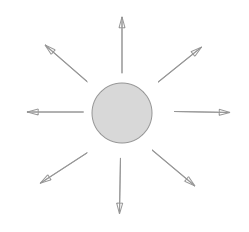Rays are emitted in all directions from the light source.

The same rule applies to our biggest light source – the sun. However, when you take into account the huge distance between the Sun and the Earth, it’s safe to treat the small percentage of rays emitted from the Sun that actually collide with Earth as parallel rays.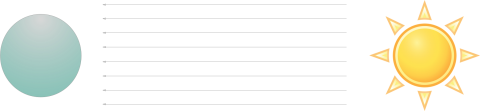For this tutorial, you’ll use only one light source with parallel rays, just like those of the sun. This is called a directional light and is commonly used in 3D games.

## Phong Lighting Model

There are various algorithms used to shade objects based on light sources, but one of the most popular is called the Phong lighting model.

This model is popular for a good reason. Not only is it quite simple to implement and understand, but it’s also quite performant and looks great!

The Phong lighting model consist of three components: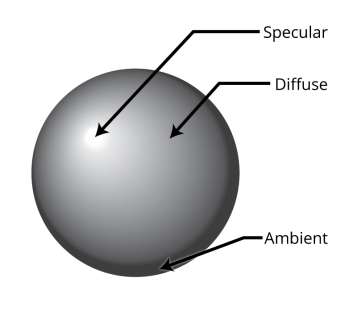1. Ambient Lighting: Represents light that hits an object from all directions. You can think of this as light bouncing around a room.
2. Diffuse Lighting: Represents light that is brighter or darker depending on the angle of an object to the light source. Of all three components, I’d argue this is the most important part for the visual effect.
3. Specular Lighting: Represents light that causes a bright spot on the small area directly facing the light source. You can think of this as a bright spot on a shiny piece of metal.

## Project Setup

It’s time to code! Start by downloading the starter project for this tutorial. It’s exactly where we finished in the previous tutorial.

Run it on a Metal-compatible iOS device, just to be sure it works correctly. You should see the following: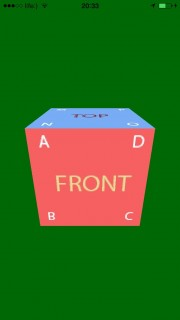This represents a 3D cube. It looks great except all areas of the cube are evenly-lit, so it looks a bit flat. You’ll improve the image through the power of lighting!

## Ambient Lighting Overview

Remember that ambient lighting highlights all surfaces in the scene by the same amount, no matter where the surface is located, which direction the surface is facing, or what the light direction is.

To calculate ambient lighting, you need two parameters:

1. Light color: Light can have different colors. For example, if a light is red, each object the light hits will be tinted red. For this tutorial, you will use a plain white color for the light. White light is a common choice, since white doesn’t tint the material of the object.
2. Ambient intensity: This is a value that represents the strength of the light. The higher the value, the brighter the illumination of the scene.

Once you have those parameters, you can calculate the ambient lighting as follows:

```Ambient color = Light color * Ambient intensity
```

Time to give this a shot in code!

First, you need a structure to store light data.

### Creating a Light Structure

Add a new Swift file to your project named Light.swift and replace its contents with the following:

```import Foundation

struct Light {

var color: (Float, Float, Float)  // 1
var ambientIntensity: Float       // 2

static func size() -> Int {       // 3
return MemoryLayout<Float>.size * 4
}

func raw() -> [Float] {
let raw = [color.0, color.1, color.2, ambientIntensity]   // 4
return raw
}
}
```

Reviewing things section-by-section:

1. This is a property that stores the light color in red, green, and blue.
2. This is a property that stores the intensity of the ambient effect.
3. This is a convenience function to get size of the `Light` structure.
4. This is a convenience function to convert the structure to an array of floats. You’ll use this and the `size()` function to send the light data to the GPU.

This is similar to `Vertex` structure that you created in Part 2 of this series.

Now open Node.swift and add the following constant to the class:

```let light = Light(color: (1.0,1.0,1.0), ambientIntensity: 0.2)
```

This creates a white light with a low intensity (0.2).

### Passing the Light Data to the GPU

Next you need to pass this light data to the GPU. You’ve already included the projection and model matrices in the uniform buffer; you’ll modify this to include the light data as well.

To do this, open Node.swift, and replace the following line in `init()`:

```self.bufferProvider = BufferProvider(device: device, inflightBuffersCount: 3, sizeOfUniformsBuffer: MemoryLayout<Float>.size * Matrix4.numberOfElements() * 2)
```

…with this code:

```let sizeOfUniformsBuffer = MemoryLayout<Float>.size * Matrix4.numberOfElements() * 2 + Light.size()
self.bufferProvider = BufferProvider(device: device, inflightBuffersCount: 3, sizeOfUniformsBuffer: sizeOfUniformsBuffer)
```

Here you increase the size of uniform buffers so that you have room for the light data.

Now in BufferProvider.swift change this method declaration:

```func nextUniformsBuffer(projectionMatrix: Matrix4, modelViewMatrix: Matrix4) -> MTLBuffer
```

…to this:

```func nextUniformsBuffer(projectionMatrix: Matrix4, modelViewMatrix: Matrix4, light: Light) -> MTLBuffer
```

Here you added an extra parameter for the light data. Now inside this same method, find these lines:

```memcpy(bufferPointer, modelViewMatrix.raw(), MemoryLayout<Float>.size * Matrix4.numberOfElements())
memcpy(bufferPointer + MemoryLayout<Float>.size*Matrix4.numberOfElements(), projectionMatrix.raw(), MemoryLayout<Float>.size*Matrix4.numberOfElements())
```

…and add this line just below:

```memcpy(bufferPointer + 2*MemoryLayout<Float>.size*Matrix4.numberOfElements(), light.raw(), Light.size())
```

With this additional `memcpy()` call, you copy light data to the uniform buffer, just as you did with with the projection and model view matrices.

### Modifying the Shaders to Accept the Light Data

Now that the data is being passed to the GPU, you need to modify your shader to use it. To do this, open Shaders.metal and add a new structure for the light data you pass just below the `VertexOut` structure:

```struct Light{
packed_float3 color;
float ambientIntensity;
};
```

Now modify the `Uniforms` structure to contain `Light`, as follows:

```struct Uniforms{
float4x4 modelMatrix;
float4x4 projectionMatrix;
Light light;
};
```

At this point, you can access light data inside of the vertex shader. However, you also need this data in the fragment shader.

To do this, change the fragment shader declaration to match this:

```fragment float4 basic_fragment(VertexOut interpolated [[stage_in]],
const device Uniforms&  uniforms    [[ buffer(1) ]],
texture2d<float>  tex2D     [[ texture(0) ]],
sampler           sampler2D [[ sampler(0) ]])
```

This adds the uniform data as the second parameter.

Open Node.swift. Inside `render(_:pipelineState:drawable:parentModelViewMatrix:projectionMatrix:clearColor:)`, find this line:

```renderEncoder.setVertexBuffer(uniformBuffer, offset: 0, at: 1)
```

…and add this line directly underneath:

```renderEncoder.setFragmentBuffer(uniformBuffer, offset: 0, at: 1)
```

By adding this code, you pass uniform buffer as a parameter not only to the vertex shader, but to the fragment shader as well.

While you’re in this method, you’ll notice an error on this line:

```let uniformBuffer = bufferProvider.nextUniformsBuffer(projectionMatrix, modelViewMatrix: nodeModelMatrix)
```

To fix this error, you need to pass the light data to the buffer provider. To do this, replace the above line with the following:

```let uniformBuffer = bufferProvider.nextUniformsBuffer(projectionMatrix: projectionMatrix, modelViewMatrix: nodeModelMatrix, light: light)
```

Take a step back to make sure you understand what you’ve done so far. At this point, you’ve passed lighting data from the CPU to the GPU, and more specifically, to the fragment shader. This is very similar to how you passed matrices to the GPU in previous parts of this tutorial.

Make sure you understand the flow, because you will pass some more data later in a similar fashion.

### Adding the Ambient Light Calculation

```// Ambient
Light light = uniforms.light;
float4 ambientColor = float4(light.color * light.ambientIntensity, 1);
```

This retrieves the light data from the uniforms and uses the values to calculate the `ambientColor` using the algorithm discussed earlier.

Now that you have calculated `ambientColor`, replace the last line of the method as follows:

```return color * ambientColor;
```

This multiplies the color of the material by the calculated ambient color.

That’s it! Build and run the app and you’ll see the following: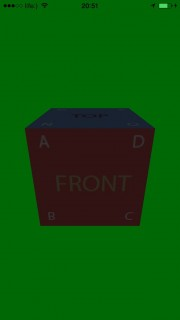### Left in the Dark

Your scene looks terribly dark now. Is this really the way ambient light is supposed to work?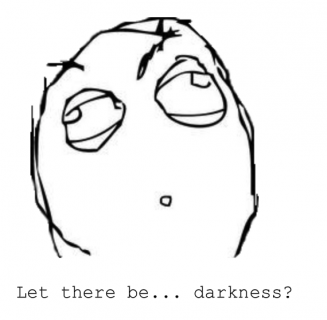Although it may seem strange, the answer is “Yes”!

Another way of looking at it is that without any light, everything would be pitch black. By adding a small amount of ambient light, you have highlighted your objects slightly, as in the early pre-dawn light.

But why hasn’t the background changed? The answer for that is simple: The vertex shader runs on all scene geometry, but the background is not geometry. In fact, it’s not even a background, it’s just a constant color which the GPU uses for places where nothing is drawn.

The green color, despite being the quintessence of awesomeness, doesn’t quite cut it anymore.

Find the following line in Node.swift inside `render(_:pipelineState:drawable:parentModelViewMatrix:projectionMatrix:clearColor:)`:

```renderPassDescriptor.colorAttachments.clearColor = MTLClearColor(red: 0.0, green: 104.0/255.0, blue: 5.0/255.0, alpha: 1.0)
```

…and replace it with the following:

```renderPassDescriptor.colorAttachments.clearColor = MTLClearColor(red: 0.0, green: 0.0, blue: 0.0, alpha: 1.0)
```

Build and run, and you’ll see the following: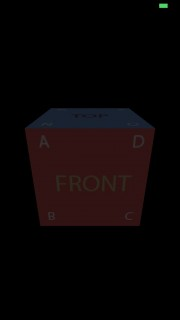Now it looks a lot less confusing!

## Diffuse Lighting Overview

To calculate diffuse lighting, you need to know which direction each vertex faces. You do this by associating a normal with each vertex.

### Introducing Normals

So what is a normal? It’s a vector perpendicular to the surface the vertex is a part of.

Take a look at this picture to see what we’re talking about:You will store the normal of each vertex in the `Vertex` structure, much like how you store texture coordinates or position values.

### Introducing Dot Products

When you’re talking about normals, you can’t escape talking about the dot product of vectors.

The dot product is a mathematical function between two vectors, such that:

• When the vectors are parallel: The dot product is equal to 1.
• When the vectors are opposite directions: The dot product of them is equal to -1.
• When the angle between the vectors is 90°: The dot product is equal to 0.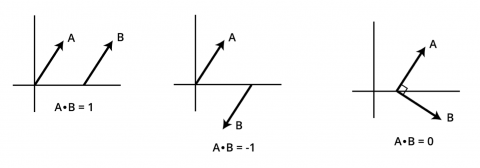This will come in handy shortly.

### Introducing Diffuse Lighting

Now that you have normals and you understand the dot product, you can turn your attention to implementing diffuse lighting.

Remember that diffuse lighting is brighter if the normal of a vector is facing toward the light, and weaker the more the normal is tilted away from it.

To calculate diffuse lighting, you need two parameters:

1. Light color: You need the color of the light, similar to ambient lighting. In this tutorial, you’ll use the same color for all types of light (ambient, diffuse, and specular).
2. Diffuse intensity: This is a value similar to ambient intensity; the bigger it is, the stronger the diffuse effect will be.
3. Diffuse factor: This is the dot product of the light direction vector and vertex normal. The smaller the angle between those two vectors, the higher this value, and the stronger the diffuse lighting effect should be.

You can calculate the diffuse lighting as follows:

```Diffuse Color = Light Color * Diffuse Intensity * Diffuse factor
```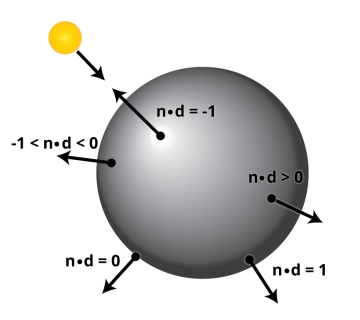In the image above, you can see the dot products of various points of the object; this represents the diffuse factor. The higher the diffuse factor, the brighter the diffuse light.

With all that theory out of the way, it’s time to dive into the implementation!

First things first; you need to add the normal data to `Vertex`.

Open Vertex.swift and find these properties:

```var s,t: Float       // texture coordinates
```

Below those properties, add the following properties:

```var nX,nY,nZ: Float  // normal
```

Now modify `func floatBuffer()` to look like this:

```func floatBuffer() -> [Float] {
return [x,y,z,r,g,b,a,s,t,nX,nY,nZ]
}
```

This adds the new normal properties to the buffer of floats.

Now open Cube.swift and change the vertices to match those:

```//Front
let A = Vertex(x: -1.0, y:   1.0, z:   1.0, r:  1.0, g:  0.0, b:  0.0, a:  1.0, s: 0.25, t: 0.25, nX: 0.0, nY: 0.0, nZ: 1.0)
let B = Vertex(x: -1.0, y:  -1.0, z:   1.0, r:  0.0, g:  1.0, b:  0.0, a:  1.0, s: 0.25, t: 0.50, nX: 0.0, nY: 0.0, nZ: 1.0)
let C = Vertex(x:  1.0, y:  -1.0, z:   1.0, r:  0.0, g:  0.0, b:  1.0, a:  1.0, s: 0.50, t: 0.50, nX: 0.0, nY: 0.0, nZ: 1.0)
let D = Vertex(x:  1.0, y:   1.0, z:   1.0, r:  0.1, g:  0.6, b:  0.4, a:  1.0, s: 0.50, t: 0.25, nX: 0.0, nY: 0.0, nZ: 1.0)

//Left
let E = Vertex(x: -1.0, y:   1.0, z:  -1.0, r:  1.0, g:  0.0, b:  0.0, a:  1.0, s: 0.00, t: 0.25, nX: -1.0, nY: 0.0, nZ: 0.0)
let F = Vertex(x: -1.0, y:  -1.0, z:  -1.0, r:  0.0, g:  1.0, b:  0.0, a:  1.0, s: 0.00, t: 0.50, nX: -1.0, nY: 0.0, nZ: 0.0)
let G = Vertex(x: -1.0, y:  -1.0, z:   1.0, r:  0.0, g:  0.0, b:  1.0, a:  1.0, s: 0.25, t: 0.50, nX: -1.0, nY: 0.0, nZ: 0.0)
let H = Vertex(x: -1.0, y:   1.0, z:   1.0, r:  0.1, g:  0.6, b:  0.4, a:  1.0, s: 0.25, t: 0.25, nX: -1.0, nY: 0.0, nZ: 0.0)

//Right
let I = Vertex(x:  1.0, y:   1.0, z:   1.0, r:  1.0, g:  0.0, b:  0.0, a:  1.0, s: 0.50, t: 0.25, nX: 1.0, nY: 0.0, nZ: 0.0)
let J = Vertex(x:  1.0, y:  -1.0, z:   1.0, r:  0.0, g:  1.0, b:  0.0, a:  1.0, s: 0.50, t: 0.50, nX: 1.0, nY: 0.0, nZ: 0.0)
let K = Vertex(x:  1.0, y:  -1.0, z:  -1.0, r:  0.0, g:  0.0, b:  1.0, a:  1.0, s: 0.75, t: 0.50, nX: 1.0, nY: 0.0, nZ: 0.0)
let L = Vertex(x:  1.0, y:   1.0, z:  -1.0, r:  0.1, g:  0.6, b:  0.4, a:  1.0, s: 0.75, t: 0.25, nX: 1.0, nY: 0.0, nZ: 0.0)

//Top
let M = Vertex(x: -1.0, y:   1.0, z:  -1.0, r:  1.0, g:  0.0, b:  0.0, a:  1.0, s: 0.25, t: 0.00, nX: 0.0, nY: 1.0, nZ: 0.0)
let N = Vertex(x: -1.0, y:   1.0, z:   1.0, r:  0.0, g:  1.0, b:  0.0, a:  1.0, s: 0.25, t: 0.25, nX: 0.0, nY: 1.0, nZ: 0.0)
let O = Vertex(x:  1.0, y:   1.0, z:   1.0, r:  0.0, g:  0.0, b:  1.0, a:  1.0, s: 0.50, t: 0.25, nX: 0.0, nY: 1.0, nZ: 0.0)
let P = Vertex(x:  1.0, y:   1.0, z:  -1.0, r:  0.1, g:  0.6, b:  0.4, a:  1.0, s: 0.50, t: 0.00, nX: 0.0, nY: 1.0, nZ: 0.0)

//Bot
let Q = Vertex(x: -1.0, y:  -1.0, z:   1.0, r:  1.0, g:  0.0, b:  0.0, a:  1.0, s: 0.25, t: 0.50, nX: 0.0, nY: -1.0, nZ: 0.0)
let R = Vertex(x: -1.0, y:  -1.0, z:  -1.0, r:  0.0, g:  1.0, b:  0.0, a:  1.0, s: 0.25, t: 0.75, nX: 0.0, nY: -1.0, nZ: 0.0)
let S = Vertex(x:  1.0, y:  -1.0, z:  -1.0, r:  0.0, g:  0.0, b:  1.0, a:  1.0, s: 0.50, t: 0.75, nX: 0.0, nY: -1.0, nZ: 0.0)
let T = Vertex(x:  1.0, y:  -1.0, z:   1.0, r:  0.1, g:  0.6, b:  0.4, a:  1.0, s: 0.50, t: 0.50, nX: 0.0, nY: -1.0, nZ: 0.0)

//Back
let U = Vertex(x:  1.0, y:   1.0, z:  -1.0, r:  1.0, g:  0.0, b:  0.0, a:  1.0, s: 0.75, t: 0.25, nX: 0.0, nY: 0.0, nZ: -1.0)
let V = Vertex(x:  1.0, y:  -1.0, z:  -1.0, r:  0.0, g:  1.0, b:  0.0, a:  1.0, s: 0.75, t: 0.50, nX: 0.0, nY: 0.0, nZ: -1.0)
let W = Vertex(x: -1.0, y:  -1.0, z:  -1.0, r:  0.0, g:  0.0, b:  1.0, a:  1.0, s: 1.00, t: 0.50, nX: 0.0, nY: 0.0, nZ: -1.0)
let X = Vertex(x: -1.0, y:   1.0, z:  -1.0, r:  0.1, g:  0.6, b:  0.4, a:  1.0, s: 1.00, t: 0.25, nX: 0.0, nY: 0.0, nZ: -1.0)
```

This adds a normal to each vertex.

If you don’t understand those normal values, try sketching a cube on a piece of paper. For each vertex, write its normal vertex value. You will get the same numbers as me!

It makes sense that all vertices on the same face should have the same normal values.

Build and run, and you’ll see the following: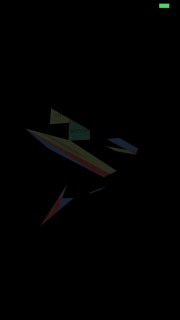Woooooooooooow! If epic glitches like this aren’t a good reason to learn 3D graphics, then what is? :]

Do you have any idea what went wrong?

### Passing the Normal Data to the GPU

At this point your vertex structure includes normal data, but your shader isn’t expecting this data yet!

Therefore, the shader reads position data for next vertex where normal data from the previous vertex is stored. That’s why you end up with this weird glitch.

To fix this, open Shaders.metal. In `VertexIn` structure, add this below all the other components:

```packed_float3 normal;
```

Build and run. Voilà — the cube looks just like expected.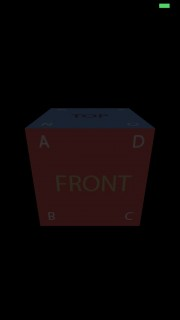Right now, your `Light` structures don’t have all the data they need for diffuse lighting. You’ll have to add some.

In Shaders.metal, add two new values to the bottom of the `Light` structure:

```packed_float3 direction;
float diffuseIntensity;
```

Now open Light.swift and add these properties below `ambientIntensity`:

```var direction: (Float, Float, Float)
var diffuseIntensity: Float
```

Also modify both methods to look like the following:

```static func size() -> Int {
return MemoryLayout<Float>.size * 8
}

func raw() -> [Float] {
let raw = [color.0, color.1, color.2, ambientIntensity, direction.0, direction.1, direction.2, diffuseIntensity]
return raw
}
```

You’ve simply added two properties, used those properties when getting the raw float array and increased the size value.

Next open `Node.swift` and modify the light constant to match this:

```let light = Light(color: (1.0,1.0,1.0), ambientIntensity: 0.2, direction: (0.0, 0.0, 1.0), diffuseIntensity: 0.8)
```

The direction that you pass (0.0, 0.0, 1.0) is a vector perpendicular to the screen. This mean that the light is pointing in the same direction as the camera. You also set the diffuse intensity to a large amount (0.8), because this is meant to represent a strong light shining on the cube.

### Adding the Diffuse Lighting Calculation

Now you can actually use the normal data. Right now you have normal data in the vertex shader, but you need the interpolated normal for each fragment. So you need to pass the normal data to `VertexOut`.

To do this, open Shaders.metal and add the following below the other components inside `VertexOut` :

```float3 normal;
```

In the vertex shader, find this line:

```VertexOut.texCoord = VertexIn.texCoord;
```

```VertexOut.normal = (mv_Matrix * float4(VertexIn.normal, 0.0)).xyz;
```

This way you will get the normal value for each fragment in a fragment shader.

Now in the fragment shader, add this right after the ambient color part:

```//Diffuse
float diffuseFactor = max(0.0,dot(interpolated.normal, light.direction)); // 1
float4 diffuseColor = float4(light.color * light.diffuseIntensity * diffuseFactor ,1.0); // 2
```

Taking each numbered comment in turn:

1. Here you calculate the diffuse factor. There is some math involved here. From right to left:
1. You take the dot product of the fragment normal and the light direction.
2. As discussed previously, this will return values from -1 to 1, depending on the angle between the two normals.
3. You need this value to be capped from 0 to 1, so you use `max` to normalize any negative values to 0.
2. To get the diffuse color, you multiply the light color with the diffuse intensity and the diffuse factor. You also set `alpha` to 0 and make it a `float4` value.

You’re nearly done! Change the last line in the fragment shader from this:

```return color * ambientColor;
```

…to this:

```return color * (ambientColor + diffuseColor);
```

Build and run, and you’ll see the following: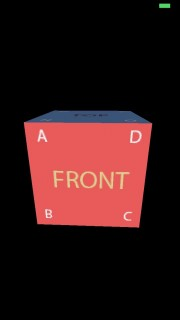Looking good, eh? For an even better look, find this line in Node.swift:

```let light = Light(color: (1.0,1.0,1.0), ambientIntensity: 0.2, direction: (0.0, 0.0, 1.0), diffuseIntensity: 0.8)
```

And change the ambient intensity to 0.1:

```let light = Light(color: (1.0,1.0,1.0), ambientIntensity: 0.1, direction: (0.0, 0.0, 1.0), diffuseIntensity: 0.8)
```

Build and run again, and there will be less ambient light, making the diffuse effect more noticeable: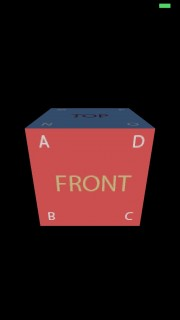As you can see, the more the face is pointed toward the light source, the brighter it becomes;## Specular Lighting Overview

Specular lighting is the third and final component of the Phong lighting model.

Remember, you can think of this component as the one that exposes the shininess of objects. Think of a shiny metallic object under a bright light: you can see a small, shiny spot.

You calculate the specular color in a similar way as the diffuse color:

```SpecularColor = LightColor * SpecularIntensity * SpecularFactor
```

Just like diffuse and ambient intensity, you can modify the specular intensity to get the “perfect” look you’re going for.

But what is the specular factor? Take a look at the following picture: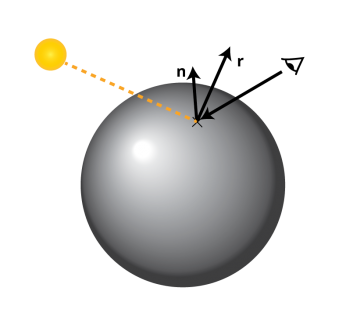This illustration shows a light ray hitting a vertex. The vertex has a normal (n), and the light reflects off the vertex in a particular direction (r). The question is: how close is that reflection vector to the vector that points toward the camera?

1. The more this reflected vector points towards the camera, the more shiny you want this point to be.
2. The farther this vector is from the camera, the darker the fragment should become. Unlike diffuse lighting, you want this dropoff effect to happen fairly quickly, to get this cool metallic effect.

To calculate the specular factor, you use your good old buddy, the dot product:

```SpecularFactor = - (r * eye)shininess
```

After you get the dot product of the reflected vector and the eye vector, you raise it to a new value – “shininess”. Shininess is a material parameter. For example, wooden objects will have less “shininess” than metallic objects.

First things first: open Light.swift and add two properties below the others:

```var shininess: Float
var specularIntensity: Float
```
Note: Shininess is not a parameter of light, it’s more like a parameter of the object material. But for the sake of this tutorial, you will keep it simple and pass it with the light data.

As always, don’t forget to modify the methods to include the new values:

```static func size() -> Int {
return MemoryLayout<Float>.size * 10
}

func raw() -> [Float] {
let raw = [color.0, color.1, color.2, ambientIntensity, direction.0, direction.1, direction.2, diffuseIntensity, shininess, specularIntensity]
return raw
}
```

In Node.swift, change the light constant value to this:

```let light = Light(color: (1.0,1.0,1.0), ambientIntensity: 0.1, direction: (0.0, 0.0, 1.0), diffuseIntensity: 0.8, shininess: 10, specularIntensity: 2)
```

Now open Shaders.metal and add this to its `Light` structure:

```float shininess;
float specularIntensity;
```

Build and run…Crash?! Time to dig in and figure out what went wrong.

### Byte Alignment

The problem you faced is a bit complicated. In your `Light` structure, `size` returns MemoryLayout.size * 10 = 40 bytes.

In `Shaders.metal`, your `Light` structure should also be 40 bytes, because that’s exactly the same structure. Right?

Yes — but that’s not how the GPU works. The GPU operates with memory chunks 16 bytes in size..

Replace the `Light` structure in Shaders.metal with this:

```struct Light{
packed_float3 color;      // 0 - 2
float ambientIntensity;          // 3
packed_float3 direction;  // 4 - 6
float diffuseIntensity;   // 7
float shininess;          // 8
float specularIntensity;  // 9

/*
_______________________
|0 1 2 3|4 5 6 7|8 9    |
-----------------------
|       |       |       |
| chunk0| chunk1| chunk2|
*/
};
```

Even though you have 10 floats, the GPU is still allocating memory for 12 floats — which gives you a mismatch error.

To fix this crash, you need to increase the `Light` structure size to match those 3 chunks (12 floats).

Open Light.swift and change `size()` to return 12 instead of 10:

```static func size() -> Int {
return MemoryLayout<Float>.size * 12
}
```

Build and run. Everything should work as expected:### Adding the Specular Lighting Calculation

Now that you’re passing the data through, it’s time for the calculation itself.

Open Shaders.metal, and add the following value to the `VertexOut` struct, right below `position`:

```float3 fragmentPosition;
```

In the vertex shader, find this line:

```VertexOut.position = proj_Matrix * mv_Matrix * float4(VertexIn.position,1);
```

…and add this line right below it:

```VertexOut.fragmentPosition = (mv_Matrix * float4(VertexIn.position,1)).xyz;
```

This new “fragment position” value does just what it says: it’s a fragment position related to a camera. You’ll use this value to get the eye vector.

Now add the following under the diffuse calculations in the fragment shader:

```//Specular
float3 eye = normalize(interpolated.fragmentPosition); //1
float3 reflection = reflect(light.direction, interpolated.normal); // 2
float specularFactor = pow(max(0.0, dot(reflection, eye)), light.shininess); //3
float4 specularColor = float4(light.color * light.specularIntensity * specularFactor ,1.0);//4
```

This is the same algorithm you learned about earlier:

1. Get the eye vector.
2. Calculate the reflection vector of the light across the current fragment.
3. Calculate the specular factor.
4. Combine all the values above to get the specular color.

Now with modify the `return` line in the fragment shader to match the following:

```return color * (ambientColor + diffuseColor + specularColor);
```

Build and run.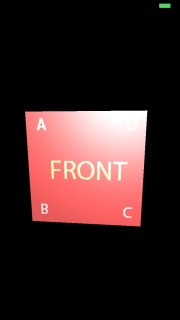## Where to Go From Here?

Here is the final example project from this iOS Metal Tutorial.

Nicely done! Take a moment to review what you’ve done in this tutorial:

1. You created a Light structure to send with matrices in a uniform buffer to the GPU.
2. You modified the BufferProvider class to handle Light data.
3. You implemented ambient lighting, diffuse lighting, and specular lighting.
4. You learned how the GPU handles memory, and fixed your crash.

Go for a walk, take a nap or play around with your app a little — you totally deserve some rest! :]

Don’t feel tired? Then feel free to check out some of these great resources:

You also might enjoy the Beginning Metal course on our site, where we explain these same concepts in video form, but with even more detail.

Thank you for joining me for this tour through Metal. As you can see, it’s a powerful technology that’s relatively easy to implement — once you understand how it works!

If you have questions, comments or Metal discoveries to share, please leave them in the comments below!